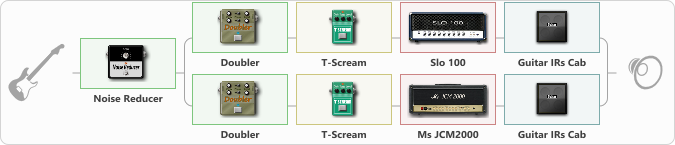# Rhythm variation

Discussion in 'ToneLib-GFX presets' started by tod, Feb 11, 2020.

1. Rhythm variation

Preset name: Rhythm variation

bridge EMG pick-up

Effects chain:Effect: "Noise Reducer" (Dynamics / Filter), active - "yes"
{
"Sens" = 90
"Mode" = Hard
}

Effect: "Splitter" (Dynamics / Filter), active - "yes"
{
"A-Bypass" = Off
"A-Pan" = -29
"A-Level" = 55
"B-Bypass" = Off
"B-Pan" = 27
"B-Level" = 55

Left branch:
{

Effect: "Doubler" (Dynamics / Filter), active - "yes"
{
"A-Dly" = 34
"A-Inv" = Off
"A-Level" = 82
"B-Dly" = 17
"B-Inv" = Off
"B-Level" = 39
}

Effect: "T-Scream" (Overdrive / Distortion), active - "yes"
{
"Drive" = 27
"Tone" = 72
"Level" = 63
}

Effect: "Slo 100" (Amp simulators), active - "yes"
{
"Gain" = 92
"Bass" = 89
"Middle" = 41
"Treble" = 50
"Presence" = 81
"Master" = 50
"Level (dB)" = 0
}

Effect: "Guitar IRs Cab" (Cabinets), active - "yes"
{
"Model" = Marshall 1960A (4x12")
"Mic Position" = Middle
"Mic Distance" = Near
"Low Cut (Hz)" = 60
"Hi Cut (kHz)" = 20.0
"Mix" = 100
"Level (dB)" = 0
}
}
Right branch:
{

Effect: "Doubler" (Dynamics / Filter), active - "yes"
{
"A-Dly" = 20
"A-Inv" = Off
"A-Level" = 82
"B-Dly" = 19
"B-Inv" = Off
"B-Level" = 44
}

Effect: "T-Scream" (Overdrive / Distortion), active - "yes"
{
"Drive" = 27
"Tone" = 54
"Level" = 63
}

Effect: "Ms JCM2000" (Amp simulators), active - "yes"
{
"Gain" = 100
"Bass" = 96
"Middle" = 48
"Treble" = 64
"Presence" = 72
"Master" = 55
"Level (dB)" = 0
}

Effect: "Guitar IRs Cab" (Cabinets), active - "yes"
{
"Model" = Marshall 1960b (4x12")
"Mic Position" = Middle
"Mic Distance" = Near
"Low Cut (Hz)" = 60
"Hi Cut (kHz)" = 20.0
"Mix" = 100
"Level (dB)" = 0
}
}
}

Note: You will need to download and install the ToneLib-GFX software to use the preset.

#### Attached Files:

• ###### Rhythm_variation.tlgfx
File size:
1,023 bytes
Views:
3,101
Rikhat and NickyFingers like this.